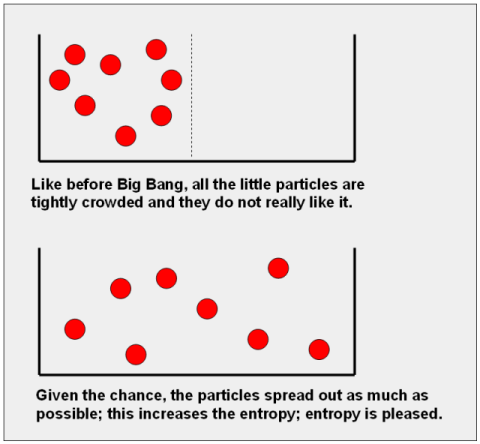Entropy (derived from a Greek word meaning “a turning towards”) in general is one of those concepts that can be used in several branches of science. Generally speaking, it is the measure by which we can determine how disordered a system is.When relating entropy to thermodynamics and Gibbs free energy, entropy determines just how unavailable the system’s energy is to do any work. In fact, entropy is so important in thermodynamics that it actually is integral to the Second Law of Thermodynamics (which states that “the entropy of an isolated system which not in a balance or equilibrium will increase over time, and would reach a maximum value at equilibrium”) and the Fundamental Thermodynamic Relation (which shows, in the form of equations, “infinitesimal change in internal energy whenever there is an infinitesimal change in entropy and change in volume for a closed system in thermal equilibrium). Both of these, of course, deal with physical processes and if they do or don’t happen unexpectedly.

In this section of the site, we will be discussing the many nuances of entropy in the context of thermodynamics. We will also be explaining the effects of entropy changes, with many simple and easy-to-understand examples for those who are new to the concept.